# 4.4 Mode-Space Transformation

A mode space approach significantly reduces the size of the HAMILTONian matrix . Due to quantum confinement along the CNT circumference, circumferential modes appear and transport can be described in terms of these modes. If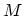modes contribute to transports, and if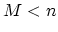, then the size of the problem is reduced from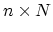to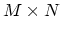, where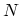is number of carbon rings along the CNT. If the potential profile does not vary sharply along the CNT, subbands are decoupled  and one can solveone-dimensional problems of size.

Mathematically, one performs a basis transformation on the HAMILTONian of the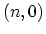zigzag CNT to decouple the problem into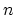one-dimensional mode space lattices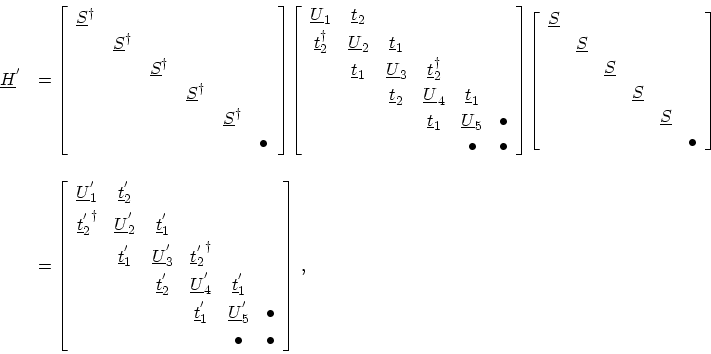(4.20)

with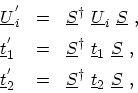(4.21)

where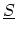is the transformation matrix from the real space basis to the mode space basis. The purpose is to decouple the modes after the basis transformation, i.e., to make the HAMILTONian matrix blocks between different modes equal to zero. This requires that after the transformation, the matrices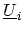,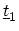, and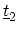, become diagonal. Sinceandare identity matrices multiplied by a constant, they remain unchanged and diagonal after any basis transformation,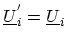and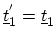. To diagonalize, elements of the transformation matrixhave to be the eigen-vectors of. These eigen-vectors are plane waves with wave-vectors satisfying the periodic boundary condition around the CNT. The eigen-values are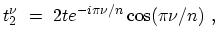(4.22)

where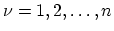. The phase factor in (4.22) has no effect on the results such as charge and current density, thus it can be omitted and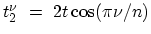can be used instead.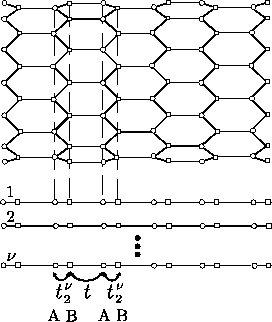After the basis transformation all sub-matrices,,, andare diagonal. By reordering the basis according to the modes, the HAMILTONian matrix takes the form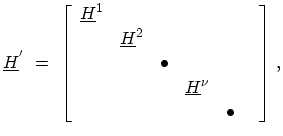(4.23)

where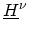is the HAMILTONian matrix for the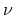th mode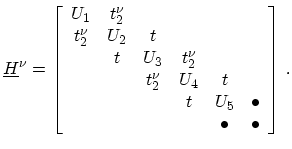(4.24)

The one-dimensional tight-binding HAMILTONian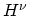describes a chain of atoms with two sites per unit cell and on-site potential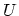and hopping parameters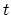and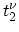(Fig. 4.7). The spatial grid used for device simulation corresponds to the circumferential rings of carbon atoms. Therefore, the rank of the matrices for each subband are equal to the total number of these rings. Self-energies can be also transformed into mode space, see Section 4.5 and Section 4.6. The GREEN's functions can therefore be defined for each subband (mode) and one can solve the system of transport equations for each subband independently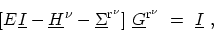(4.25)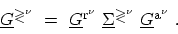(4.26)

M. Pourfath: Numerical Study of Quantum Transport in Carbon Nanotube-Based Transistors